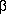V. FORMATS FOR THE IRAS SKY SURVEY ATLAS (ISSA)

# ISSA Explanatory Supplement V. FORMATS FOR THE IRAS SKY SURVEY ATLAS (ISSA)

The IRAS Sky Survey Atlas, ISSA, and the ISSA Reject Set are machine-readable images in Flexible Image Transport System (FITS) (Wells, D.C. et al. 1981) image format. Each 500 × 500-pixel image covers a 12.5° × 12.5° field of sky with pixel size of 1.5'. For each field and wavelength, intensity images were produced for separate HCONs plus the co-add of all HCONs. Coverage and standard deviation images were also produced and can be obtained from IPAC by special request.

FITS image format restrictions require that image samples be either 16 or 32 bits. The dynamic range of the intensity values in each ISSA image drove the choice of sample size. The resolution is 5% of median pixel noise. Intensity images are either 0.5 or 1.0 Mbytes. Sample FITS headers for intensity images are shown in Tables V.1 and V.2. Table V.3 provides a brief description of some FITS header keywords.

Table V.1 A Sample FITS Header for Intensity Images:
(4 byte format)
```SIMPLE  =                    T        / STANDARD FITS FORMAT
BITPIX  =                   32        / 4 byte twos-compl int
NAXIS   =                    3        / # OF AXES
NAXIS1  =                  500        / # SAMPLES PER LINE
NAXIS2  =                  500        / # LINES IN IMAGE
NAXIS3  =                    1        / # WAVELENGTHS
BSCALE  =      2.540014267E-03        / TRUE=TAPE*BSCALE+BZERO
BZERO   =      3.795739345E+02        /
BUNIT   = 'MJY/SR  '                  / INTENSITY
BLANK   =          -2000000000        / TAPE VALUE FOR EMPTY PIXEL
CRVAL1  =      1.560000000E+02        / RA AT ORIGIN (DEGREES)
CRPIX1  =      2.500000000E+02        /  SAMPLE AXIS ORIGIN (PIXEL)
CTYPE1  = 'RA---TAN'                  /  DECREASES IN VALUE AS SAMPLE
/  INDEX INCREASES (GNOMONIC)
CDELT1  =     -2.500000000E-02        /  COORD VALUE INCR DEG/PIXEL
/  AT ORIGIN ON SAMPLE AXIS
CRVAL2  =      7.000000000E+01        /  DEC AT ORIGIN (DEGREES)
CRPIX2  =      2.500000000E+02        /  LINE AXIS ORIGIN (PIXEL)
CTYPE2  = 'DEC--TAN'                  /  DECREASES IN VALUE AS LINE
/  INDEX INCREASES (GNOMONIC)
CDELT2  =      2.500000000E-02        /  COORD VALUE INCR DEG/PIXEL
/  AT ORIGIN ON LINE AXIS
CRVAL3  =      6.000000000E-05        /  WAVELENGTH IN METERS
CRPIX3  =      1.000000000E+00        /
CTYPE3  = 'LAMBDA  '                  /
CDELT3  =      0.000000000E+00        /
DATAMAX =      7.604873693E+02        /  MJY/SR (TRUE VALUE)
DATAMIN =     -1.339500327E+00        /  MJY/SR (TRUE VALUE)
EPOCH   =      1.950000000E+03        /  EME50
DATE-MAP= '91/03/11'                  /  MAP RELEASE DATE (YY/MM/DD)
DATE    = '91/10/28'                  /  DATE TAPE WRITTEN(YY/MM/DD)
ORIGIN  = 'JPL-IPAC'                  /  INSTITUTION
TELESCOP= 'IRAS    '                  /
INSTRUME= 'ISSA-FLD'                  /  IRAS SKY SURVEY ATLAS
OBJECT  = 'f414h003'                  /  FIELD NUMBER-HCON
PROJTYPE= 'GNOMONIC'                  /  PROJECTION TYPE
EDITED  =                    T        /  SCANS EDITED
APPCAL  =                    T        /  CALIBRATION CORRECTION 25 MICRON
DE-ZODY =                    T        /  DE-ZODIED IMAGE
GLBL-D  =                    T        /  APPLIED GLOBAL PARAMETERS
LOCAL-D =                    T        /  LOCAL DESTRIPER
ASBLANK =                    T        /  ASTEROID BLANKING
COMMENT                               /
COMMENT
COMMENT
COMMENT
COMMENT
COMMENT
COMMENT
END

```

Table V.2 A Sample FITS Header for Intensity Images:
(2 byte format)
```SIMPLE  =                    T        / STANDARD FITS FORMAT
BITPIX  =                   16        / 2 byte twos-compl int
NAXIS   =                    3        / # OF AXES
NAXIS1  =                  500        / # SAMPLES PER LINE
NAXIS2  =                  500        / # LINES IN IMAGE
NAXIS3  =                    1        / # WAVELENGTHS
BSCALE  =      8.622583455E-05        / TRUE=TAPE*BSCALE+BZERO
BZERO   =      2.175757902E+00        /
BUNIT   = 'MJY/SR  '                  / INTENSITY
BLANK   =               -32768        / TAPE VALUE FOR EMPTY PIXEL
CRVAL1  =      2.730000000E+02        / RA AT ORIGIN (DEGREES)
CRPIX1  =      2.500000000E+02        /  SAMPLE AXIS ORIGIN (PIXEL)
CTYPE1  = 'RA---TAN'                  /  DECREASES IN VALUE AS SAMPLE
/  INDEX INCREASES (GNOMONIC)
CDELT1  =     -2.500000000E-02        /  COORD VALUE INCR DEG/PIXEL
/  AT ORIGIN ON SAMPLE AXIS
CRVAL2  =      4.000000000E+01        /  DEC AT ORIGIN (DEGREES)
CRPIX2  =      2.500000000E+02        /  LINE AXIS ORIGIN (PIXEL)
CTYPE2  = 'DEC--TAN'                  /  DECREASES IN VALUE AS LINE
/  INDEX INCREASES (GNOMONIC)
CDELT2  =      2.500000000E-02        /  COORD VALUE INCR DEG/PIXEL
/  AT ORIGIN ON LINE AXIS
CRVAL3  =      6.000000000E-05        /  WAVELENGTH IN METERS
CRPIX3  =      1.000000000E+00        /
CTYPE3  = 'LAMBDA  '                  /
CDELT3  =      0.000000000E+00        /
DATAMAX =      4.978097525E+00        /  MJY/SR (TRUE VALUE)
DATAMIN =     -6.265817208E-01        /  MJY/SR (TRUE VALUE)
EPOCH   =      1.950000000E+03        /  EME50
DATE-MAP= '91/05/15'                  /  MAP RELEASE DATE (YY/MM/DD)
DATE    = '91/10/28'                  /  DATE TAPE WRITTEN(YY/MM/DD)
ORIGIN  = 'JPL-IPAC'                  /  INSTITUTION
TELESCOP= 'IRAS    '                  /
INSTRUME= 'ISSA-FLD'                  /  IRAS SKY SURVEY ATLAS
OBJECT  = 'f358h001'                  /  FIELD NUMBER-HCON
PROJTYPE= 'GNOMONIC'                  /  PROJECTION TYPE
EDITED  =                    T        /  SCANS EDITED
APPCAL  =                    T        /  CALIBRATION CORRECTION 25 MICRON
DE-ZODY =                    T        /  DE-ZODIED IMAGE
GLBL-D  =                    T        /  APPLIED GLOBAL PARAMETERS
LOCAL-D =                    T        /  LOCAL DESTRIPER
ASBLANK =                    T        /  ASTEROID BLANKING
COMMENT                               /
COMMENT
COMMENT
COMMENT
COMMENT
COMMENT
COMMENT
END
```

Table V.3 FITS Keywords
KeywordDescription
BITPIX Indicates number of bits to represent the sample in two's complement. Intensity maps are either 16 or 32 bits per sample. Coverage and standard deviation images are 16 bits per sample.
BUNIT Intensity and standard deviation of the mean are in antom{MJy/sr} MJy sr-1. Coverage images are dimensionless.
BLANK Value assigned to empty pixels.
DATE Date the image was made at IPAC.
INSTRUME Indication that image is an IRAS Sky Survey Atlas or ISSA Reject product. ISSA-I or ISSA-FLD indicates the ISSA image was processed for the first release in 1991, the || > 50° sky. ISSA-II indicates the ISSA image was processed for the second release, the || < 50° sky. ISSA-REJ indicates the image is part of the ISSA Reject Set.
OBJECT Gives field and HCON number as fxxxhnnn where xxx is a three-digit field number and nnn is either 001,002 or 003 for individual HCON or 000 for the co-add.
CRVAL3 Wavelength in meters.
True/false indicators:
EDITED If TRUE, the field had some initial scans edited based on a list of known anomalies.
APPCAL If TRUE, calibration corrections were applied to the 25 µm detectors.
DE-ZODY If TRUE, the image has had the zodiacal foreground removed.
GLBL-D If TRUE, the image has been globally destriped.
LOCAL-D If TRUE, the image has been locally destriped.
ASBLANK If TRUE, the image has had asteroids removed from the co-added image